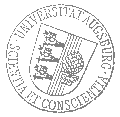# Universität AugsburgInstitut für MathematikOberseminar Numerische Mathematik

Professor Dr. Euan Spence
University of Bath

spricht am

Dienstag, 14. Januar 2020

um

16:00 Uhr

im

Raum 1008 (L1)

über das Thema:

## »For most frequencies, strong trapping has a weak effect in frequency-domain scattering«

 Abstract: It is well known that when the geometry and/or coefficients allow stable trapped rays, the solution operator of the Helmholtz equation grows exponentially through a sequence of real frequencies tending to infinity. In this talk (based on the paper https://arxiv.org/abs/1903.12172 ) we show that, even in the presence of the strongest- possible trapping, if a set of frequencies of arbitrarily small measure is excluded, the Helmholtz solution operator grows at most polynomially as the frequency tends to infinity. One significance application of this result is in the convergence analysis of several numerical methods for solving the Helmholtz equation at high frequency that are based on a polynomial- growth assumption on the solution operator (e.g. hp-finite elements, hp-boundary elements, certain multiscale methods). The result of this talk shows that this assumption holds, even in the presence of the strongest-possible trapping, for most frequencies.

 Hierzu ergeht herzliche Einladung. Prof. Dr. Daniel Peterseim

[Impressum]      [Datenschutz]      wwwadm@math.uni-augsburg.de,     Mo 13-Jan-2020 16:55:01 MEZ